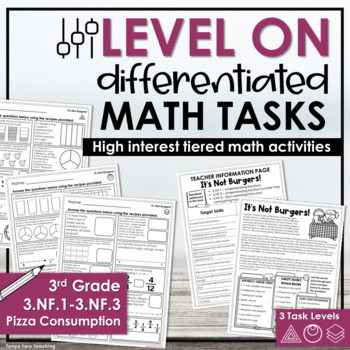EASEL BY TPT

Total:
\$0.00# 3rd Grade Differentiated Math Worksheets Fractions 3.NF.1 - 3.NF.33rd, Homeschool
Subjects
Standards
Resource Type
Formats Included
• PDF
Pages
12 pages
\$3.99
\$3.99

#### Also included in

1. Level On Differentiated Math Tasks are ready to print math tasks with high interest topics. The questions build in complexity and are tiered so you can differentiate your classroom based on student ability. How is this resource differentiated? There are 3 printables of questions. Each page represen
\$18.00
\$35.91
Save \$17.91

### Description

Level On Differentiated Math Tasks are ready to print math tasks with high interest topics. The questions build in complexity and are tiered so you can differentiate your classroom based on student ability.

How is this resource differentiated?

There are 3 printables of questions. Each page represents a level. Look for different icons at the top right corner of each page. They are meant to be discrete so students don’t know they have a different set of questions.

BENEFITS OF THIS RESOURCE:

• Saves you valuable time- no more piecing together resources
• Low prep- just print and go
• Differentiate to 3 ability levels at once
• Symmetry in your instruction- all 3 levels share a common passage and layout

HOW CAN I USE THIS RESOURCE?

• Unit review/ standard review
• Math Test Prep
• Homework
• Classroom/ extra practice
• Math rotations
• PBL assignments

WHAT'S INCLUDED IN THIS RESOURCE?

Information Sheet for Students– Each one of our Level On Differentiated Math Tasks starts with an introduction about a particular topic. These topics were selected based on student interest and are appropriate for the grade level the math curriculum is connected to. EVERY student gets this page despite which set of questions they are assigned to.

Pages with Math Questions– Pick and choose which pages each student should complete. There are 3 pages that each have 10 questions each. All questions are written with conceptual understanding in mind. They are meant to stretch student thinking.

Standards and topics covered in this Level On Math Task:

• 3.NF.1 – Understanding fractions
• 3.NF.2 – Understanding fractions on number lines
• 3.NF.3 – Equivalent fractions and comparting fractions
Total Pages
12 pages
Included
Teaching Duration
1 hour
Report this Resource to TpT
Reported resources will be reviewed by our team. Report this resource to let us know if this resource violates TpT’s content guidelines.

### Standards

to see state-specific standards (only available in the US).
Understand a fraction 1/𝘣 as the quantity formed by 1 part when a whole is partitioned into 𝘣 equal parts; understand a fraction 𝘢/𝑏 as the quantity formed by 𝘢 parts of size 1/𝘣.
Understand a fraction as a number on the number line; represent fractions on a number line diagram.
Represent a fraction 1/𝘣 on a number line diagram by defining the interval from 0 to 1 as the whole and partitioning it into 𝘣 equal parts. Recognize that each part has size 1/𝘣 and that the endpoint of the part based at 0 locates the number 1/𝘣 on the number line.
Represent a fraction 𝘢/𝘣 on a number line diagram by marking off 𝘢 lengths 1/𝘣 from 0. Recognize that the resulting interval has size 𝘢/𝘣 and that its endpoint locates the number 𝘢/𝘣 on the number line.
Explain equivalence of fractions in special cases, and compare fractions by reasoning about their size.